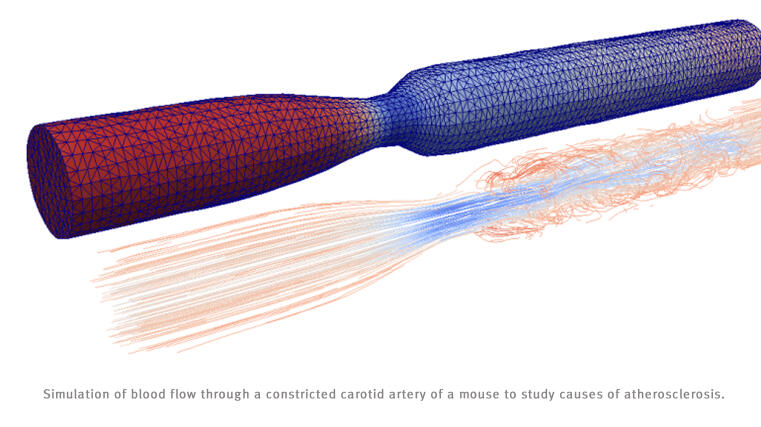© WWU/FB10

Applied Mathematics deals with the development of analytical and numerical mathematical concepts and methods that can (potentially) be applied to treat questions from other scientific disciplines. This includes mathematical modelling of problems in the life, natural, and engineering sciences and the analytical and/or numerical treatment of the resulting, usually nonlinear, systems of differential equations and optimization problems. Also the efficient implementation for computer simulations is covered.

From the mathematical perspective, particularly methods from analysis (theory of partial differential equations, functional analysis, theory of dynamical systems, regularity analysis, calculus of variations, ...), methods from numerics (discretization methods, convergence analysis, a priori and a posteriori error estimation, model reduction, ...) as well as the development and mathematical analysis of efficient algorithms are employed.

Depending on the focus, connections to other mathematical areas abound, e.g. differential geometry, functional analysis, and stochastics. In addition to the mathematical focus it is possible to write the thesis in close collaboration with groups from the application side, e.g. biology and medicine in Münster.

# Prerequisites

Specialisation Applied Analysis (corresponding to the specialisation "Applied Mathematics" in the old PO 2013)

Basic courses on ordinary and partial differential equations, covering:

Fundamentals of ordinary differential equations, Gauß' theorem, examples of partial differential equations, method of characteristics and differential equations of first order, elliptic partial differential equations and weak formulation, Sobolev spaces, parabolic differential equations

Specialisation Numerics and Scientific Computing (corresponding to the specialisation "Scientific Computing" in the old PO 2013)

Basic courses on numerical analysis and programming courses, covering:

Fundamentals of iterative methods to solve linear systems of equations, Newton method, fundamentals of ordinary differential equations, fundamentals of programming, a basic understanding of partial differential equations, weak and strong formulations, Gauß' theorem, Sobolev spaces

# Courses for the specialisation in Applied Analysis (AA) and Numerics and Scientific Computing (SC)

Winter semester 2020/2021

JProf. Dr. Manuel Friedrich: Partial Differential Equations (Type I, AA)
Prof. Dr. Arnulf Jentzen: Machine Learning for Partial Differential Equations, in German (Type I, SC)
Prof. Dr. Carsten Wolters: Modern Applied Mathematics in Bioelectromagnetism (Type I or II, AA, SC), 2+1 SWS, continuation in the summer semester 2021
Dr. Frank Wübbeling: Inverse Problems (Type I, AA)
Pro.f Dr. Caterina Zeppieri: Calculus of Variations (Type I, AA)

Summer semester 2021

Prof. Dr. Gustav Holzegel: Non-linear Wave Equations (Type I, AA)
Dr. Benno Kuckuck: Mathematical Introduction to Deep Learning (Type I, II, SC)
Prof. Dr. Mario Ohlberger: Numerical Methods for Partial Differential Equations II (Type I, SC)
Prof. Dr. André Schlichting: Stochastic Control Theory (Type I, AA)
Prof. Dr. Christian Seis: Introduction to Mathematical Fluid Dynamics (Type I, AA)
Prof. Dr. Angela Stevens: Special Topics in PDE (PDE III) (Type I, AA)
Prof. Dr. Benedikt Wirth: Mathematical Optimization, in German (Type I, AA, SC)
Prof. Dr. Carsten Wolters: Modern Applied Mathematics in Bioelectromagnetism (Type I or II, AA, SC), 2+1 SWS, continuation of the winter semester 2020/20201

Winter semester 2021/2022

Prof. Dr. Manuel Friedrich: Fine properties of Sobolev functions (Type I, AA)
Prof. Dr. André Schlichting: Longtime behaviour of dynamic systems (Type I, AA)
Prof. Dr. Benedikt Wirth: Inverse Problems, in German (Type I, AA, SC)
Prof. Dr. Carsten Wolters: Mathematics in Bioelectromagnetism (Type I or II, SC)

# Seminars

Winter semester 2020/2021

Prof. Dr. Christian Engwer: Scientific Computing (SC)
JProf. Dr. Manuel Friedrich: Variational Methods and Modelling (AA)
Prof. Dr. Arnulf Jentzen: Mathematics of Deep Learning (SC)
Prof. Dr. Christian Seis: Mathematical Fluid Dynamics (AA)
Prof. Dr. Benedikt Wirth: Mathematical Optimization (AA, SC)
Dr. Frank Wübbeling: Inverse Problems and Medical Imaging (SC)
Prof. Dr. Caterina Zeppieri: Advanced Topics in the Calculus of Variations (AA)

Summer semester 2021

Prof. Dr. Christian Engwer: Scientific Computing (SC)
Prof. Dr. Manuel Friedrich: Variational Methods and Modelling (AA)
Dr. Benno Kuckuck: Mathematics of Deep Lerning (SC)
Prof. Dr. André Schlichting: Dynamics of complex systems (AA)
Prof. Dr. Christian Seis: Mathematical Fluid Dynamics (AA)
Prof. Dr. Angela Stevens: Analysis and Mathematical Modelling (AA)
Prof. Dr. Benedikt Wirth: Mathematical Optimaztion (AA, SC)
Dr. Frank Wübbeling: Inverse Problems and Medical Imaging (AA, SC)
Prof. Dr. Caterina Zeppieri: Advanced Topics in the Calculus of Variations (AA)

Winter semester 2021/2022

Prof. Dr. Christian Engwer: Scientific Computing (SC)
Prof. Dr. Manuel Friedrich: Variational Methods and Modelling (AA)
Prof. Dr. André Schlichting: Dynamics of complex systems (AA)
Prof. Dr. Angela Stevens: Analysis and Mathematical Modelling (AA)
Prof. Dr. Benedikt Wirth: Mathematical Optimaztion (AA, SC)
Dr. Frank Wübbeling: Inverse Problems and Medical Imaging (AA, SC)
Prof. Dr. Caterina Zeppieri: Advanced Topics in the Calculus of Variations (AA)

Seminars for AA and SC are always courses of type II. The seminars in both specialisations can in principle also be used as "first course" in the module "Specialisation Supplement and Research Skills".

# Practical courses

Winter semester 2020/2021

To be announced.

Summer semester 2021

To be announced.

Practical courses for AM and SC are always courses of type II.

# Further information

Each research group has a different focus

On the webpages of these research groups you will find further information about lectures, seminars, master theses etc.